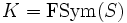# Finitary symmetric group equals center of symmetric group modulo finitary alternating group

## Statement

Let$S$ be an infinite set,$G = \operatorname{Sym}(S)$ be the Symmetric group (?) on$S$,$K = \operatorname{FSym}(S)$ be the Finitary symmetric group (?), and$H$ be the Finitary alternating group (?). Then,$K/H$ is the Center (?) of$G/H$.

## Proof

We need to pove that if$\sigma \in G$ is such that$[G,\sigma] \le H$, then$\sigma \in K$. We do this by picking any$\sigma \notin K$, and show that$[G,\sigma]$ is not contained in$H$.

If$\sigma \notin K$,$\sigma$ must move infinitely many elements. There are two cases, that are collectively exhaustive:

•$\sigma$ contains infinitely many finite cycles.
•$\sigma$ contains an infinite cycle.